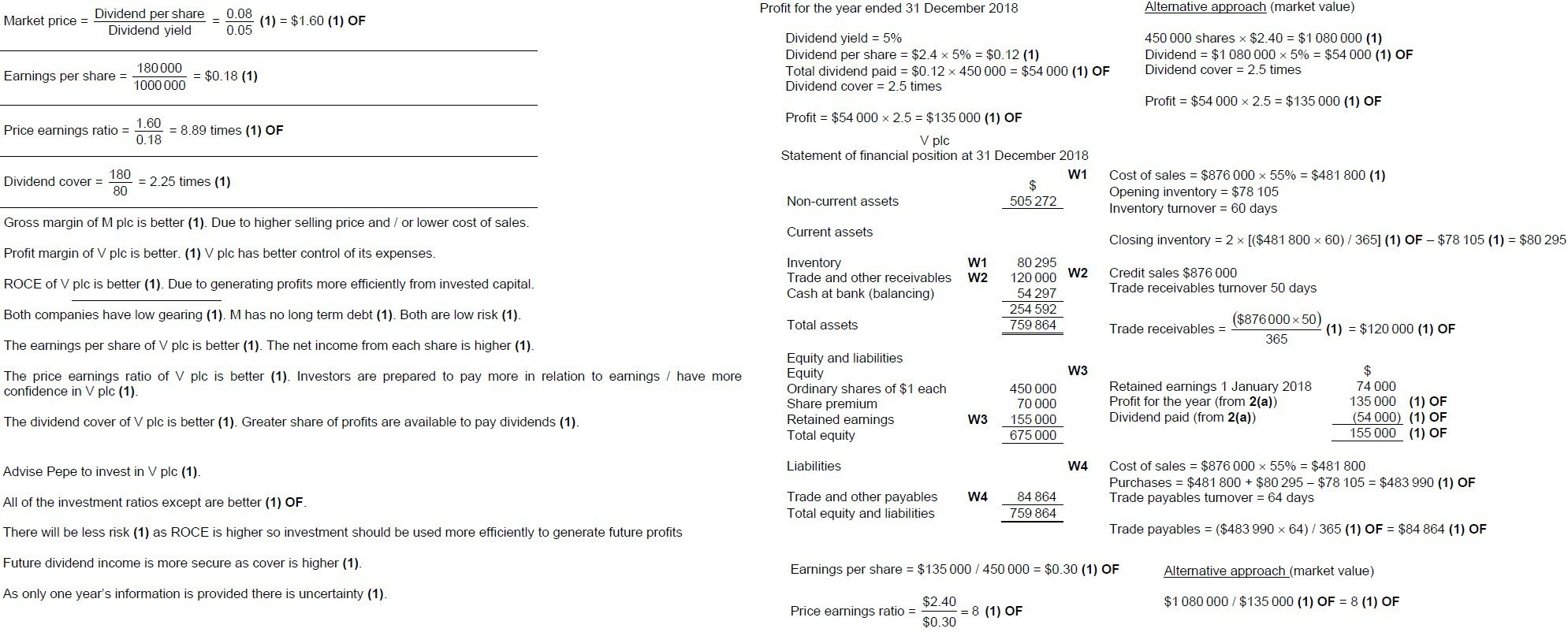Earnings per share (EPS)

EPS shows how much profit after interest (finance cost), tax, and preference dividend is attributable to shareholders.  Investors usually regard EPS as a convenient measure of the success of a company.  EPS is a tool used by potential investors to gauge the profitability of a company before buying its shares. It is calculated as follows:

EPS                  = Profit after interest, tax and preference dividend / Number of ordinary shares

Price earnings ratio (PER)

PER relates the market price of a share to the EPS. It may be regarded as the number of years earnings that investors are prepared to pay for in the purchase price of a company’s shares. The higher the PER, the greater is the confidence of investors in the company’s ability to maintain the EPS. It is calculated as follows:

PER                  =          Market price of a share / Earnings per share

=          MPS / EPS

Dividend yield

Shareholders invest in a company in order to earn dividend. They also hope that the market price of the share will rise so that when they sell the shares they make a capital profit. DY expresses the actual dividend received as a percentage of the market price of a share. It shows the actual percentage return an investor can expect based on the current market price of a share. It is of more interest to investors than dividend expressed as a percentage of the nominal value of each share or the number of cents per share. It is calculated as follows:

Dividend Yield            =          (Dividend per share / Market price of a share)*100

=          (DPS / MPS)*100

Dividend cover

Dividend cover shows how many times the ordinary dividend can be paid out of profit available to equity holders.  A low dividend cover might indicate that future ordinary dividend is at risk if profitability declines. A high dividend cover suggests that the company should be able to maintain the same rate of dividend to ordinary shareholders even if profit falls. A high dividend cover also indicates that the directors operate a conservative dividend policy and that much of the company’s profits are being reinvested in the company.

Dividend cover          =          Profit after interest, tax and preference dividend / Ordinary         dividend paid

Income gearing

This measures the proportion of profit that is being used to pay interest (finance cost). The higher is the ratio, the greater is the chance to decrease profit and deteriorate liquidity position of a business. It is calculated as follow:

Income gearing        =       (Finance cost / Operating profit) * 100

Interest cover

This is an alternative to income gearing and represents the number of times finance cost (interest) is covered by profit. The higher is the interest cover the greater is the probability for interest payment. It is calculated as follows:

Interest cover            =          Operating profit / Finance cost

Gearing ratio

This measure the fixed cost capital (debentures and PSC) of the company as a percentage of capital employed. It measures the risk of investing in the business. If the gearing ratio is greater than 50% the company is said to be a highly geared company (risky to invest). Highly geared company may cause greater risk to ordinary shareholders because fixed cost capital must be rewarded before equity holders.  It is calculated as follows:

Gearing ratio           =          (Fixed cost capital / Capital employed) * 100

=          [(PSC + Debentures) / (OSC + Reserves + Debentures + PSC) ] * 100

Question 1 and 2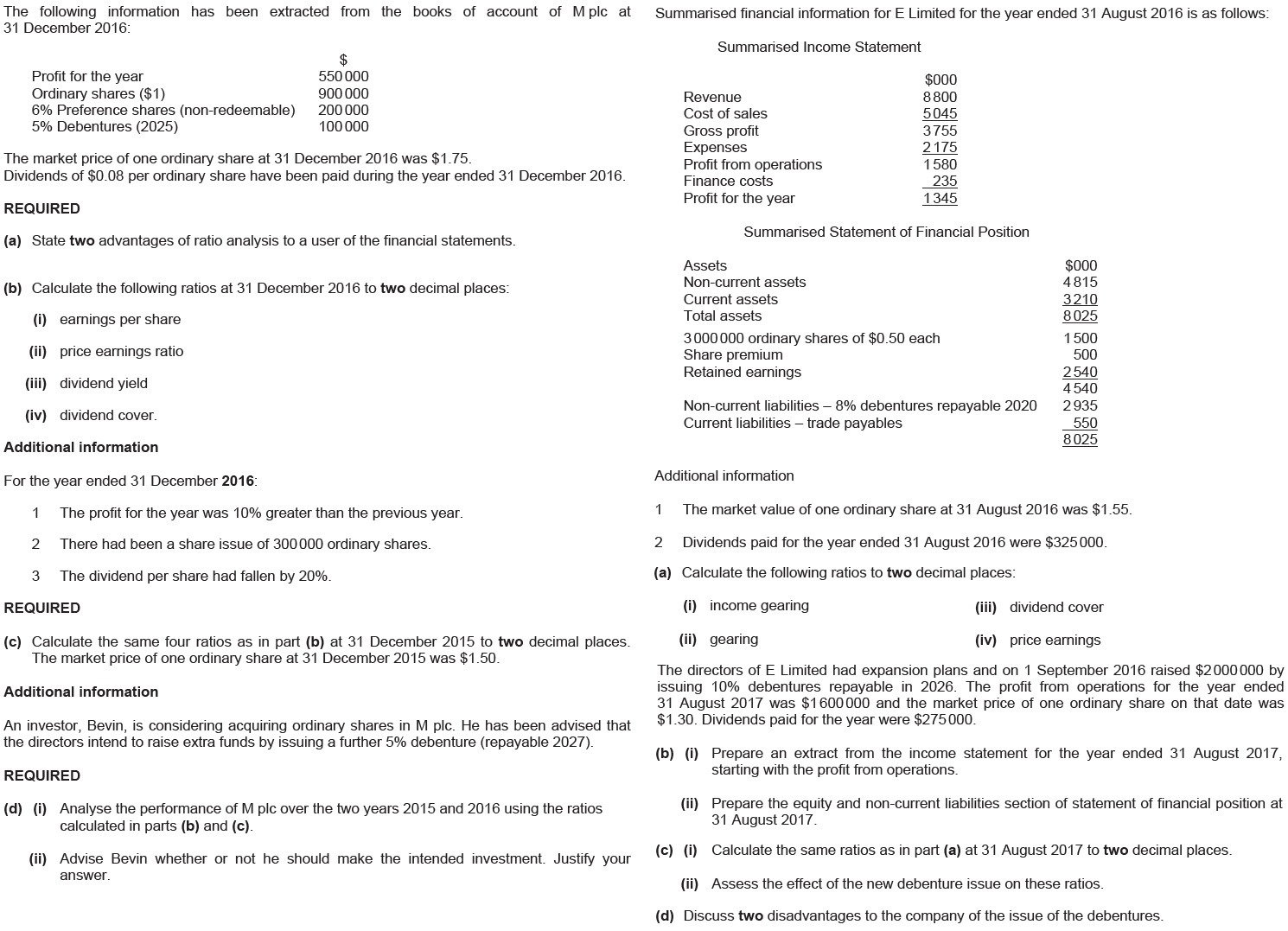Answer Question 1 and 2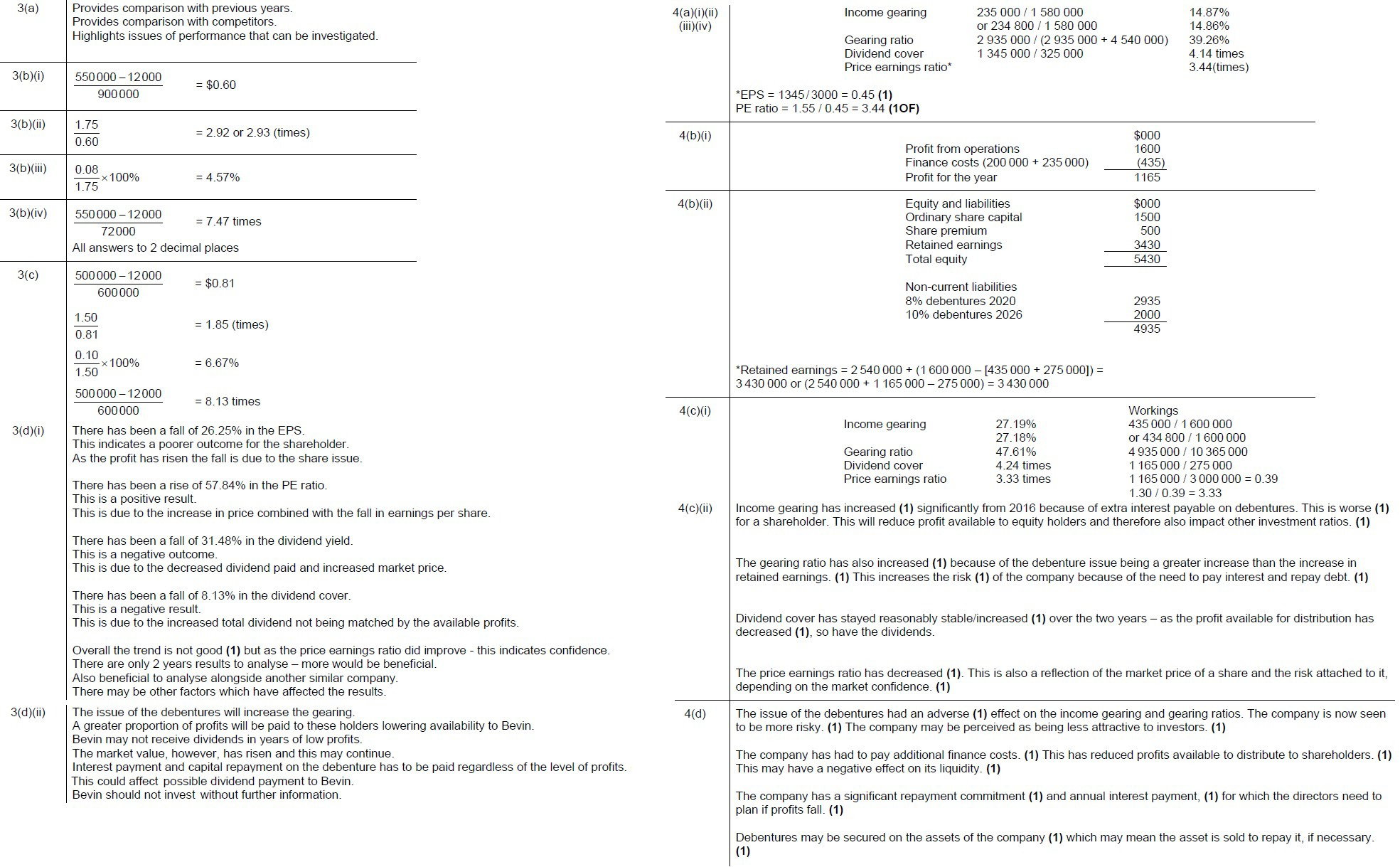Question 3 and 4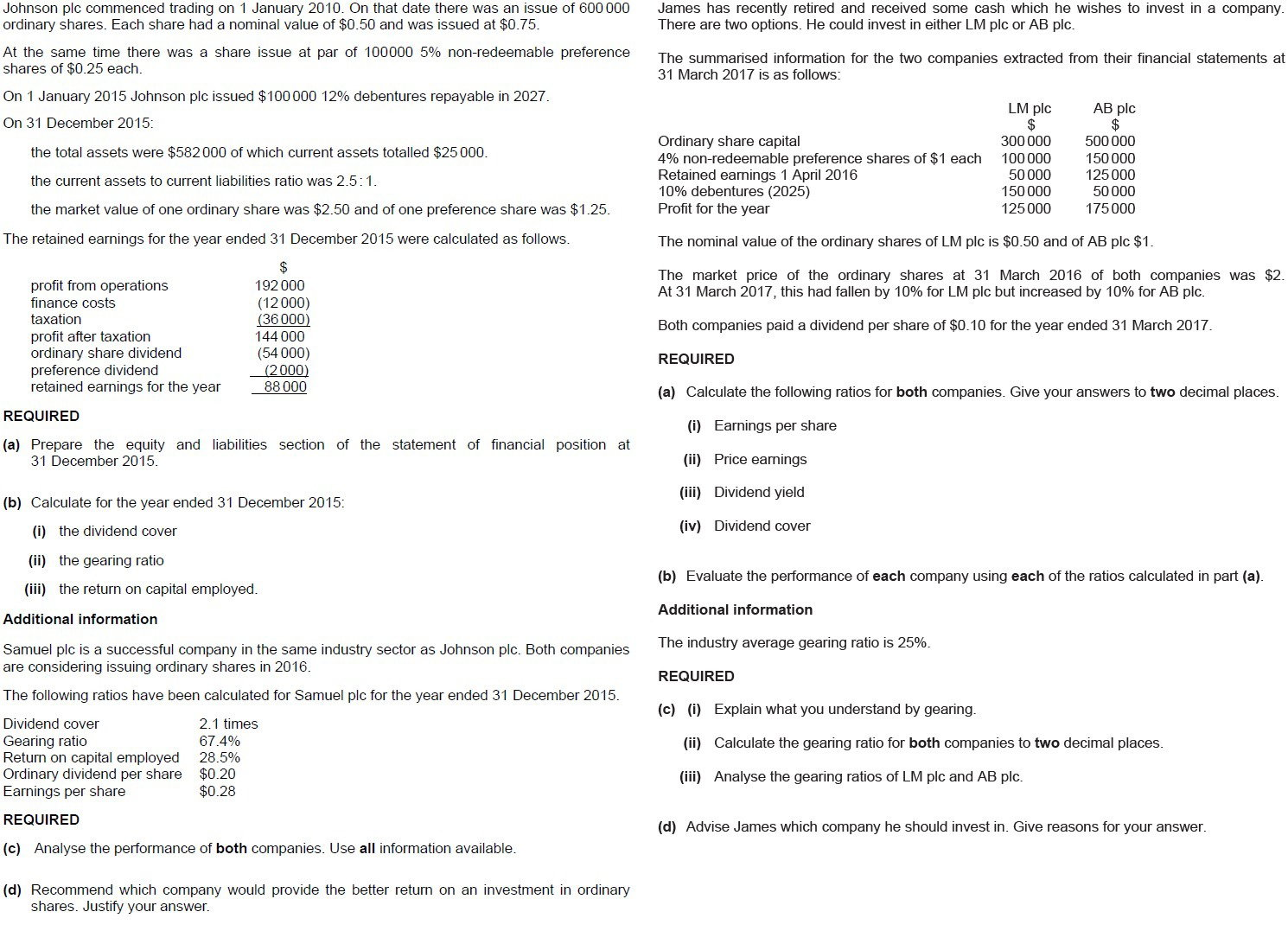Answer Question 3 and 4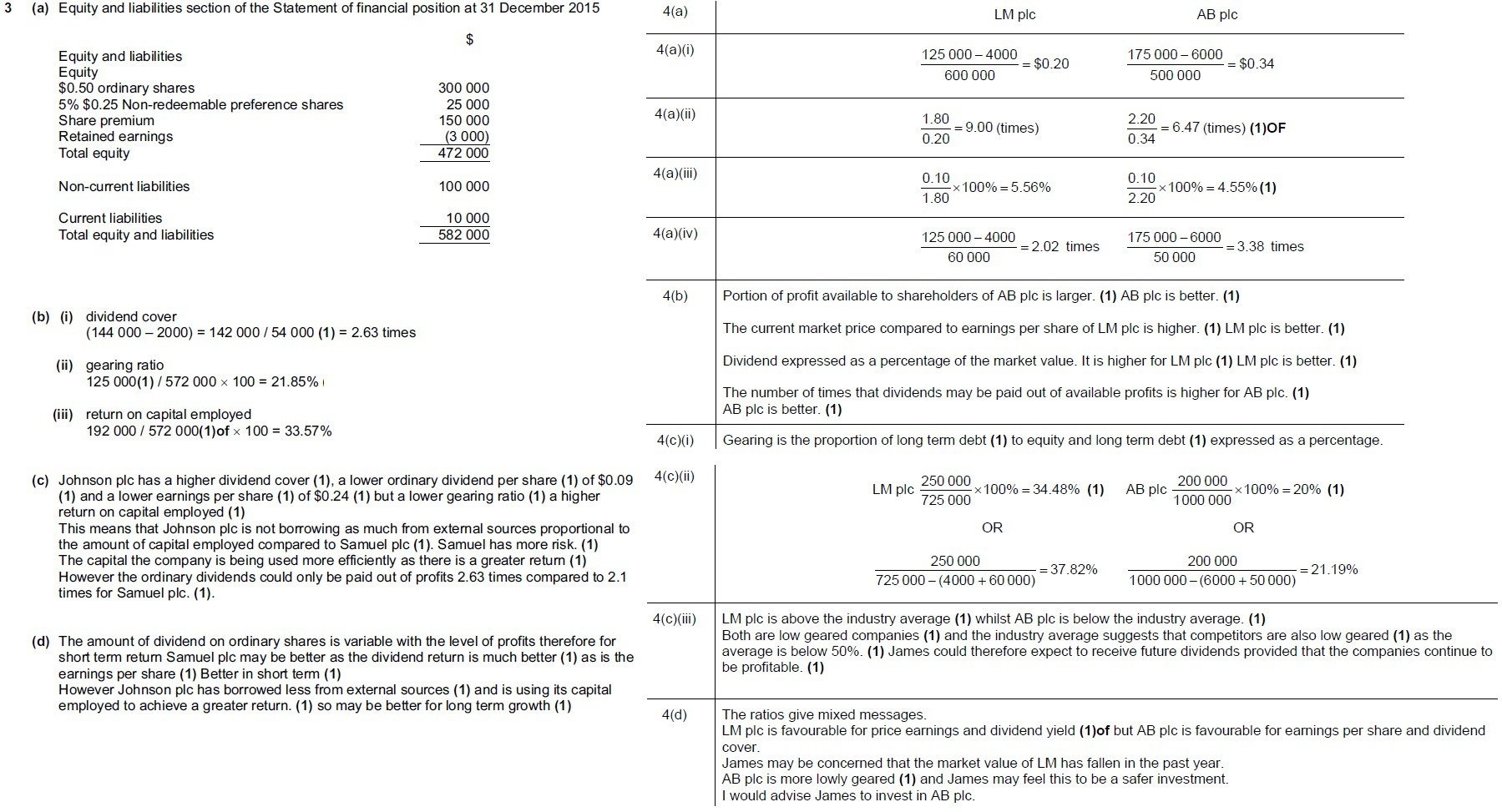Question 5 and 6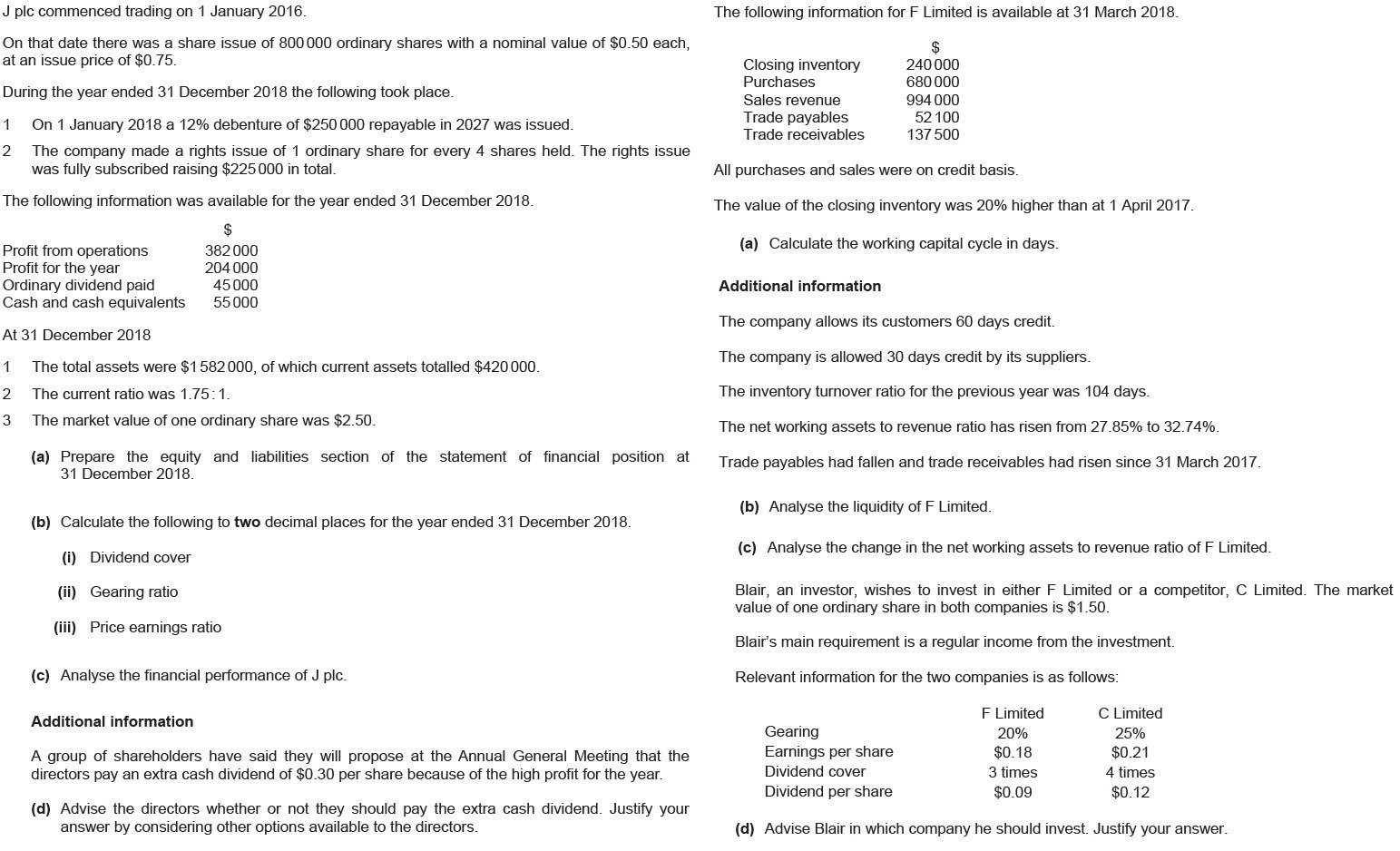Answer Question 5 and 6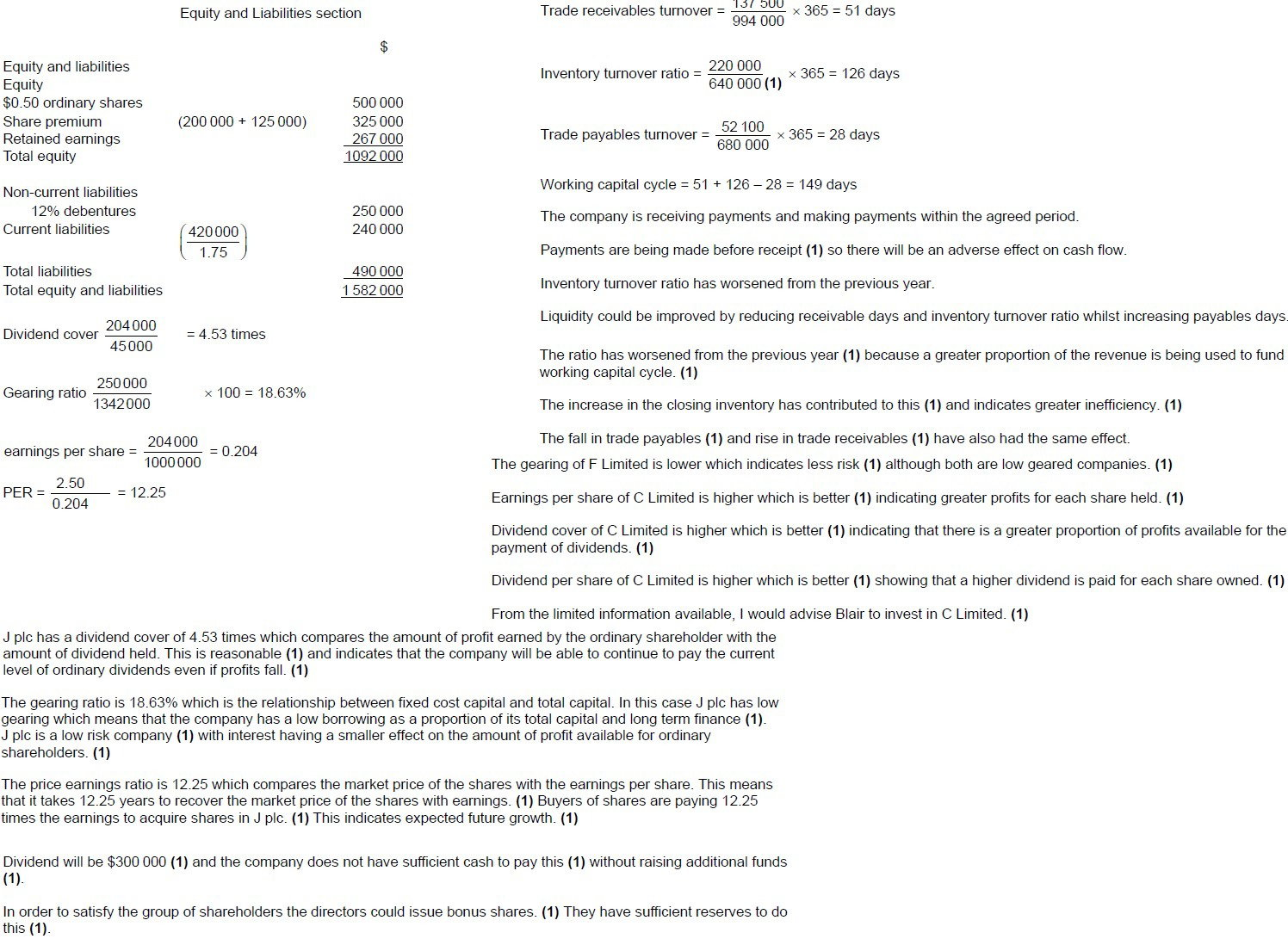Question 7 and 8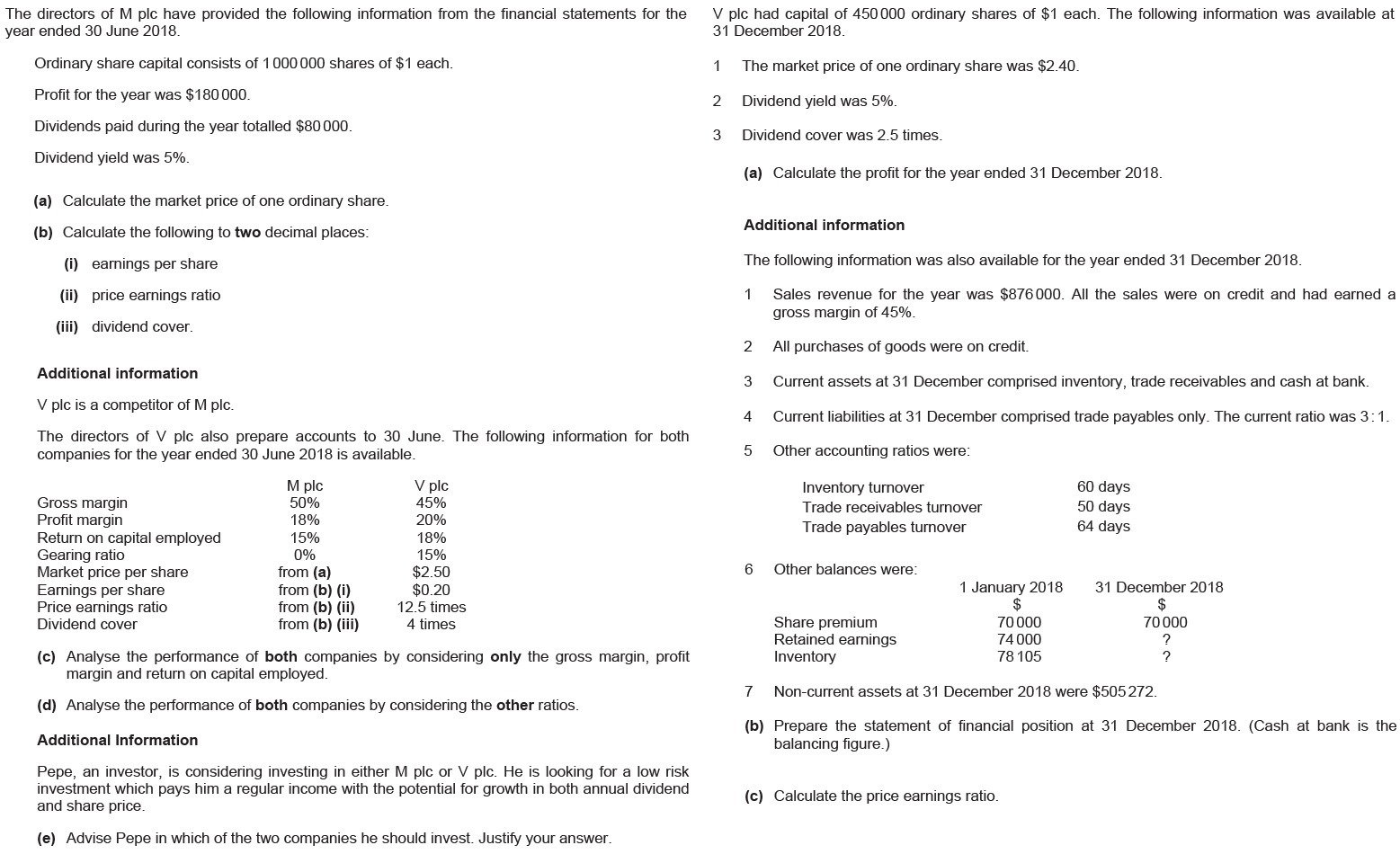Answer Question 7 and 8